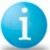# POLL: How do you calculate RTY.

Six Sigma – iSixSigma Forums Old Forums General POLL: How do you calculate RTY.

Viewing 7 posts - 1 through 7 (of 7 total)
• Author
Posts
• #28942

POLL:  How do you calculate RTY.Do you integrate over a specific set of units, or a window of time?
I am trying to decide which is the better algorithm and wouldappreciate any comments or experiences?
Cheers,Andy
Example 1, Over units.My process has three steps:  1, 2, & 3On 1/1/2002 I start four units: A, B, C, D.I wait until all four units are concluded before calculating my RTY.
Step1              Step2      Step3IN                   A,B,C,D          B,C,D      B,C,DSCRAP           A                     BREWORK      A,B                  BOUT               B,C,D              B,C,D      C,D
FTY                3/4                  3/3            2/3FPY                2/4                  2/3            2/3RTY                                                              = 2/4 * 2/3 * 2/3
Example 2, Over time.On 1/1/2002 I start counting.On 2/1/2002 I stop counting.
Step1       Step2       Step3BOH              D,E,F       A,B,C       -IN                  H,I,J,K     D,E,F       B,C,DSCRAP          H              A             BREWORK      D              BOUT               D,E,F       B,C,D      C
FTY                3/4           3/3          1/3FPY                2/4           1/3          1/3RTY                                                    = 2/4 * 1/3 * 1/3

0
#72901

Wow! My tables got complete screwed up!  It looks like all the returns in the post window–which look like hard returns–are really soft returns.Let me try posting the tables again.-Andy

0
#72904

Example 1, Over units.
My process has three steps:  1, 2, & 3
On 1/1/2002 I start four units: A, B, C, D.
I wait until all four units are concluded before calculating my RTY.
Step1              Step2      Step3
IN                   A,B,C,D         B,C,D      B,C,D
SCRAP           A                     B
REWORK      A,B                  B
OUT               B,C,D              B,C,D      C,D
FTY                3/4                   3/3           2/3
FPY                 2/4                  2/3           2/3
RTY                                                               = 2/4 * 2/3 * 2/3
Example 2, Over time.
On 1/1/2002 I start counting.
On 2/1/2002 I stop counting.
Step1           Step2        Step3BOH               D,E,F           A,B,C        -IN                   H,I,J,K         D,E,F        B,C,DSCRAP           H                 A              BREWORK       D                 BOUT                D,E,F           B,C,D      C
FTY                3/4                3/3           1/3FPY                2/4                1/3           1/3RTY                                                            = 2/4 * 1/3 * 1/3

0
#72934

RTY is a calculation that is done to highlight the “hidden Factory” and also to break the myth manufacturers use to show yield based on final test or acceptance. It is a simple calculation utilizing the probability model to show the true process yield.
To calculate you take the first pass yield at each step in the process and multiply them together:
four units into a four step process:
Yield step )1) .5 X Yield step (2) .75 X Yield at step (3) .95 X Yield at step (4) .85 = RTY = .30
Normally what would have been reported is an 85% yield if step four was the final test or acceptance point. In reality the process yield is 30%.
This is also useful to focus six sigma initiative on the worst step in the process first then the next etc…

0
#72945

Andy,
I am with RR here.  RTY is only based on “good” product after the first pass through the process.  Anything that is scrap/rework did not make it on the first pass.  Therefore looking at your first example:  A, B, C, D enter your process.  Step 1 only C and D come out (50% yeild).  [Your example breaks down as B can not be considered passing through on first pass.]  C and D go in step 2 only C comes out (50% yield).  C goes in step 3 and C comes out (100% yield).
RTY is 50%*50%*100%=25% yield not 100% yield (last step).

0
#72964

0
#72978Member

Andy ,
I agree with Bob and Andy however in real life situations I prefer to use the following method which is based on the poissons distribution.The calculations are much easier to do.All you have to do is at each process step keep track of the defects and the total units produced.Calculate the DPU at each stage. Calculate the thoughput yield using e-dpu.
Calculate RTY as a product of the above yields.
In your example 1. Total units 4 and defects 3 Hence DPU= 0.75
In step two Total units 3 defects 1  Hence DPU=0.33
In step three Total units 3 defects 1 (2 came ok hence I am considering the 1 left as defect) DPU=0.33
Using the poissons model
RTY= 0.472*0.7189*0.7189 = 24.5%
I find this method more easy to implement.I hope this helps.